Displacement, Velocity and Acceleration

# Displacement, Velocity and Acceleration - Civil Engineering (CE)

 1 Crore+ students have signed up on EduRev. Have you?

Displacement, velocity and acceleration
The vector field, u, defined as

u = x − X,                                              (3.27)

represents the displacement field, that is the displacement of the material particles initially at X or currently at x depending on whether Lagrangian or Eulerian description is used. Thus,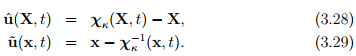Figure 3.2 schematically shows the displacement vector. As shown in the figure, displacement vector is a straight line segment joining the location of a material particle in the current and reference configuration. While, in the schematic we have used the same coordinate system to describe all the three directed line segments namely, the position vector of a particle in the reference configuration, current configuration and the displacement vector; this is not necessary. In fact, if one uses cylindrical polar coordinate basis, the position vector of the material particle in the current and reference configuration, in general would be different.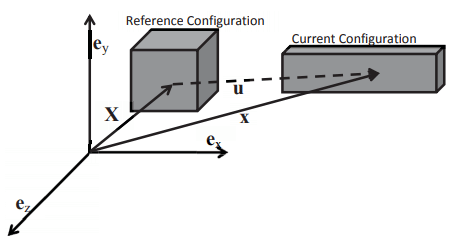Figure 3.2: Schematic of deformation of a cube (X position vector of a typical material particle in the reference configuration, x position vector of the same material particle whose position vector in the reference configuration is X in the current configuration, u displacement vector of this material particle).

The first and second total time derivatives of the motion field is called the velocity and acceleration respectively. Thus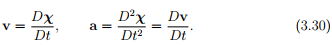We note that both the velocity and acceleration can be expressed as a Lagrangian field or Eulerian field. From the definition of the displacement (3.27) it can be seen that the velocity and acceleration can be equivalently written as,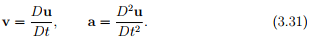As we have seen before, the displacement field can be expressed as a Lagrangian field or Eulerian field. Thus, we can have a Lagrangian displacement gradient, H and a Eulerian displacement gradient, h defined as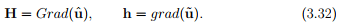Recognize that these gradients are not the same. To see this, we compute the displacement gradient in terms of the deformation gradient as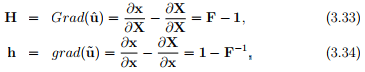Example
A certain motion of a continuum body in the material description is given in the form:

x1 = X− exp(−t)X2, x2 = exp(−t)X1 + X2, x3 = X3,            (3.35)

for t > 0. Find the displacement, velocity and acceleration components in terms of the material and spatial coordinates and time. Also find the deformation and displacement. Assume that the same Cartesian coordinate basis and origin is used to describe the body both in the current and the reference configuration.
It follows from (3.27) that

û = − exp(−t)X2E1 + exp(−t)X1E2.                                  (3.36)

Similarly, from (3.30) we obtain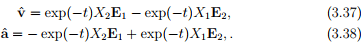Inverting the motion field (3.35) we obtain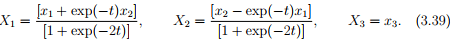Substituting (3.39) in (3.36), (3.37) and (3.38) we obtain the Eulerian form of the displacement, velocity and acceleration fields as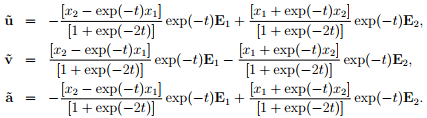Here we have made use of the fact that the same Cartesian basis vectors are used to define both the current and the reference configuration. Next, we compute the deformation gradient to be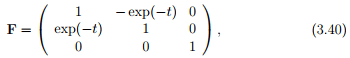and the Lagrangian and Eulerian displacement gradient as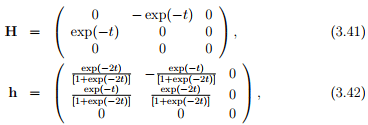Notice that at t = 0, F ≠ 1, but F tends to 1 as time, t tends to . This just indicates that the configuration chosen as reference is not the one at time t = 0, but at some other time and this is permissible.

The document Displacement, Velocity and Acceleration - Civil Engineering (CE) is a part of Civil Engineering (CE) category.
All you need of Civil Engineering (CE) at this link: Civil Engineering (CE)

Track your progress, build streaks, highlight & save important lessons and more!(Scan QR code)

,

,

,

,

,

,

,

,

,

,

,

,

,

,

,

,

,

,

,

,

,

,

,

,

;